# High School Chemistry : Identifying Limiting Reagents

## Example Questions

### Example Question #13 : Stoichiometry

Which of the following determines which reactant is the limiting reactant?

The reactant with less mass than the other reactant is the limiting reactant

The reactant taking up the least volume is the limiting reactant

The reactant with less partial pressure than the other reactant is the limiting reactant

The reactant with fewer moles than the other reactant is the limiting reactant

The reactant that cannot fully convert the other reactant is the limiting reactant

The reactant that cannot fully convert the other reactant is the limiting reactant

Explanation:

In a chemical reaction, the limiting reactant determines how much product can be created. Given two reactants in different quantities, the limiting reactant is defined as the reactants that cannot fully convert the given amount of the other reactant to product.

The mass, number of moles, volume, and partial pressure of the reactants can help to identify the limiting reagent, but cannot be used without a given chemical equation with molar ratios. For example, imagine that fifty moles of reactant A are needed to react two moles of reactant B. You are given 49 moles of reactant A and 2 moles of reactant B. Even though you have more mass, volume, and moles of reactant A, it is still the limiting reactant because it cannot fully convert reactant B to the product.

### Example Question #1 : Identifying Limiting Reagents

Consider the following reaction: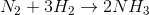If the reaction starts initially with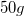of nitrogen gas and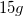of hydrogen gas, what will be the limiting reactant?

Both will run out at the same time

Hydrogen gas

Ammonia

Nitrogen gas

Nitrogen gas

Explanation:

In order to determine the limiting reactant, we can use a calculation to determine how much of one reactant is needed in order to use up the other. For example, we can see how much hydrogen gas is necessary in order to use up allof nitrogen gas that we have: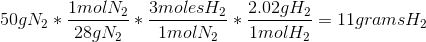In other words, we need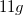of hydrogen gas in order to use upof nitrogen gas. Since we haveof hydrogen gas, we have more than enough to react all of the nitrogen gas, and the nitrogen gas will be used up before the hydrogen gas. As a result, nitrogen gas is the limiting reactant.

### Example Question #2 : Identifying Limiting Reagents

What property will be most important in determining the limiting reagent of the following reaction, given the available amount of each reactant?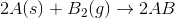Temperature of the reactants

Volume ratio of A to B

Molar ratio of A to B

Gram ratio of A to B

Pressure ratio of A to B

Molar ratio of A to B

Explanation:

Limiting reactants are determined when there is an excess of a particular reactant, in relation to the other reactants available. When reactants are compared, one must always compare the molar ratio in order to determine which reactant is limited. In this reaction, if we are given the available amount of each reactant, we will need to convert the given amount of one reactant (A) to the necessary amount of the other (B) required to fully react. If we find that reactant A is available in excess, then reactant B will be the limiting reagent. The only way to compare these two terms, however, is by using the molar ratio in the reaction.

### Example Question #4 : Identifying Limiting Reagents

Hydrogen gas combines with oxygen gas during combustion to produce water according to the chemical reaction: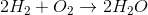If 64 grams of oxygen gas and 6 grams of hydrogen gas are combusted, what amount of reactants will you have after the reaction?

0g of hydrogen gas and 16g of oxygen gas

2g of hydrogen gas and 0g of oxygen gas

2g of hydrogen gas and 16g of oxygen gas

0g of hydrogen gas and 0g of oxygen gas

0g of hydrogen gas and 32g of oxygen gas

0g of hydrogen gas and 16g of oxygen gas

Explanation:

Let's begin by converting our reactants from grams to moles. Note that both gases are diatomic, meaning that there are two atoms per molecule.

Molecular weights: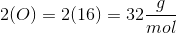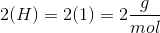Conversion to moles: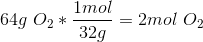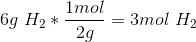We have 2 moles of oxygen gas and 3 moles of hydrogen gas. Next, look at the given reaction:Two moles of hydrogen gas are consumed to react each mole of oxygen gas. We can use this ratio to find the number of moles of oxygen gas needed to react all of the hydrogen given in this question.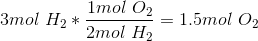Only 1.5 moles of oxygen gas are needed to react all of the hydrogen gas. Since we have 2 moles of oxygen gas, some of it will remain unreacted when all of the hydrogen gas has been used. This means the hydrogen gas is the limiting reagent.

Find the amount of unreacted oxygen gas: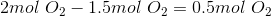Convert to grams: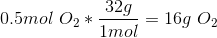### Example Question #5 : Identifying Limiting Reagents

Consider the reaction of potassium carbonate with calcium nitrate to form potassium nitrate and calcium carbonate: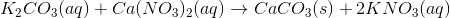Suppose 50ml of a 0.250M potassium carbonate solution was mixed with 100ml of a 0.175M calcium nitrate solution. What is the maximum amount of calcium carbonate that could be obtained?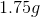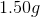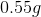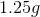Explanation:

First, we must determine how many moles of each reactant begin the reaction by multiplying the molarity by the volume. Don't forget to convert volume to liters!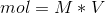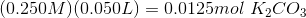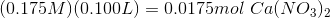Next, use the reaction coefficients (i.e. the stoichiometry) to determine how many moles of calcium carbonate could be formed from each of the reactants. In this case, there is a 1:1 molar ratio between both reactants and calcium carbonate.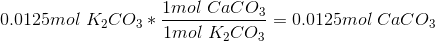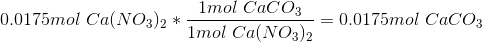Thus, 0.0125 moles of potassium carbonate could form 0.0125 moles of calcium carbonate, while 0.0175 moles of calcium nitrate could form 0.0175 moles of calcium carbonate. The maximum amount of product is going to be determined by the limiting reactant, i.e. the reactant that provides the least amount of product. In this case, the limiting reactant is potassium carbonate, and the maximum yield of calcium carbonate is 0.0125mol.

For the final step convert this value to grams: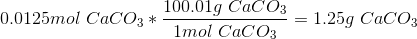### Example Question #6 : Identifying Limiting Reagents

Consider the reaction of potassium carbonate with calcium nitrate to form potassium nitrate and calcium carbonate:Suppose 50ml of a 0.250M potassium carbonate solution was mixed with 100ml of a 0.175M calcium nitrate solution. What is the maximum amount of calcium carbonate that could be obtained?Explanation:

First, we must determine how many moles of each reactant begin the reaction by multiplying the molarity by the volume. Don't forget to convert volume to liters!Next, use the reaction coefficients (i.e. the stoichiometry) to determine how many moles of calcium carbonate could be formed from each of the reactants. In this case, there is a 1:1 molar ratio between both reactants and calcium carbonate.Thus, 0.0125 moles of potassium carbonate could form 0.0125 moles of calcium carbonate, while 0.0175 moles of calcium nitrate could form 0.0175 moles of calcium carbonate. The maximum amount of product is going to be determined by the limiting reactant, i.e. the reactant that provides the least amount of product. In this case, the limiting reactant is potassium carbonate, and the maximum yield of calcium carbonate is 0.0125mol.

For the final step convert this value to grams:### All High School Chemistry Resources